# Moment of inertia avoid double integral?

• Pascal1p

## Homework Statement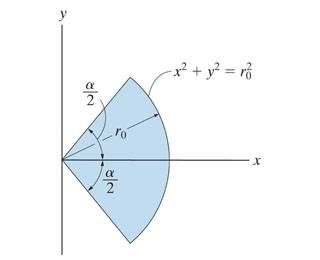Determine the moment of inertia of the shaded area about the x-axis.

I(x)= y^2dA

## The Attempt at a Solution

In order to determine the moment of inertia of the shaded area about the x-axis I first looked at the portion above the x-axis, integrate it with a small rectangular element and then because of the symmetry I would multiply it with 2. So: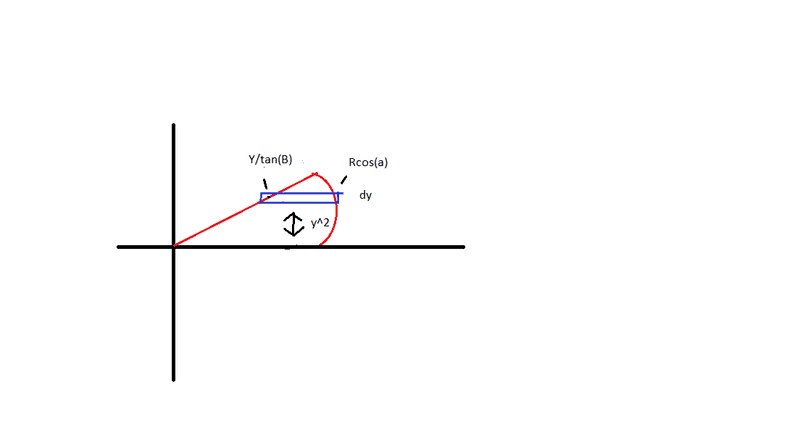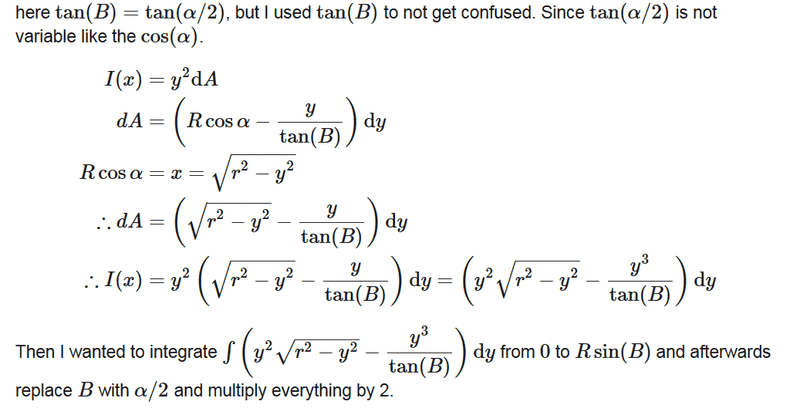Yet if I integrate that with those limits and then multiply it with 2 and insert B= a/2
I do not get (a-sin (a))(r^4)/8. The answer I would get if I just use ∫∫((r*sinθ)^2)rdθdr. Which is the correct answer.

But why doesn't it work?

But why doesn't it work?
It does. I checked. It's just that the integral is messy, so it's easy to make an error somewhere along the way.
Start with this, then apply that indefinite integral formula at the integration limits. The trick that cleans it up a lot is that
$$\tan^{-1}\left(\frac{R\sin\theta}{\sqrt{R^2-(R\sin \theta)^2)}}\right)=\theta$$

It does. I checked. It's just that the integral is messy, so it's easy to make an error somewhere along the way.
Start with this, then apply that indefinite integral formula at the integration limits. The trick that cleans it up a lot is that
$$\tan^{-1}\left(\frac{R\sin\theta}{\sqrt{R^2-(R\sin \theta)^2)}}\right)=\theta$$

But where then does this inconsistency come from? My book gives some standard integrals and one of them was ∫x^2√(a^2-x^2)dx= -(x/4)√((a^2-x^2)^3)+ (a^2/8)(x√(a^2-x^2)+a^2sin^-1 (x/a) + C, a>0

The weird thing is that wolfram-alpha gives this long answer when you substitute 'a' with an actual number, like 9. But when you use just 'a' or substitute it with 'R', it gives a different form. The one with the tan^-1 which you just mentioned.

So I thought the answer you get when you use wolfram with y^2* sqrt(R^2-y^2) is wrong. That he might see R as something it is not, like a function related to y. Instead of the constant value that it is.

So why is -(x/4)√((a^2-x^2)^3)+ (a^2/8)(x√(a^2-x^2)+a^2sin^-1 (x/a) + C not the correct solution to the integral?

The weird thing is that wolfram-alpha gives this long answer when you substitute 'a' with an actual number, like 9.
It didn't do that for me.

So why is -(x/4)√((a^2-x^2)^3)+ (a^2/8)(x√(a^2-x^2)+a^2sin^-1 (x/a) + C not the correct solution to the integral?
Because when you differentiate it you do not get x^2√(a^2-x^2).
I did this by hand and it did not come out, and Wolfram Alpha agrees. Perhaps your text contains an error, or perhaps you have miscopied the formula.

It didn't do that for me.

Because when you differentiate it you do not get x^2√(a^2-x^2).
I did this by hand and it did not come out, and Wolfram Alpha agrees. Perhaps your text contains an error, or perhaps you have miscopied the formula.

Well, the link you send, in which you said it did not do that for you. It also gives an arcsin and looked similair to what book give so I thought they were same without really checking.

I did miss a parenthesis in the end, but still what the book says does not seem the same.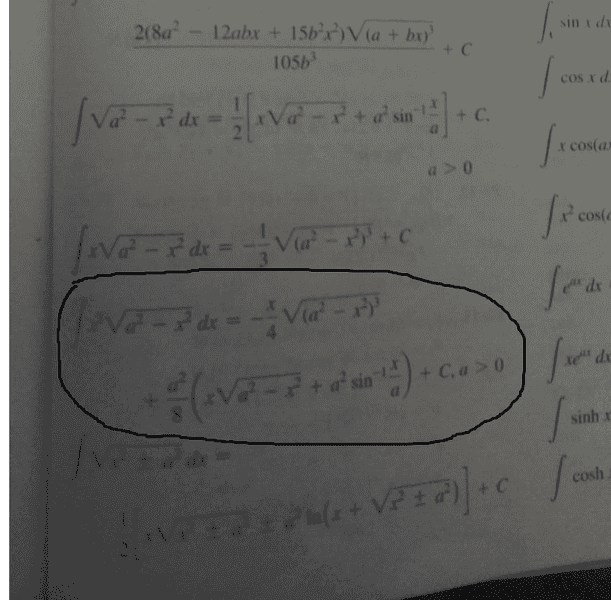Well, the link you send, in which you said it did not do that for you. It also gives an arcsin
Recall your trig identities, in particular that ##\sin^{-1}\frac xa=\tan^{-1}\frac x{\sqrt{a^2-x^2}}##
and looked similair to what book give so I thought they were same without really checking.

I did miss a parenthesis in the end
Yes, and it was the missing parenthesis that led you to thinking the book suggested a different answer.
but still what the book says does not seem the same.
It is the same. A little algebra demonstrates that. Start by taking ##\sqrt{(a^2-x^2)^2}## out of the first term in the book and combining it with the second term (the first term in the big parenthesis on the next line).

The lesson to take from this is that if you have a messy calculation that you believe should come out to a particular result and it doesn't, set your work out in full detail and check every step for things like misplaced parentheses and wrong signs. One tiny error is all that's needed to make the whole thing fail.

Recall your trig identities, in particular that ##\sin^{-1}\frac xa=\tan^{-1}\frac x{\sqrt{a^2-x^2}}##

Yes, and it was the missing parenthesis that led you to thinking the book suggested a different answer.
It is the same. A little algebra demonstrates that. Start by taking ##\sqrt{(a^2-x^2)^2}## out of the first term in the book and combining it with the second term (the first term in the big parenthesis on the next line).

The lesson to take from this is that if you have a messy calculation that you believe should come out to a particular result and it doesn't, set your work out in full detail and check every step for things like misplaced parentheses and wrong signs. One tiny error is all that's needed to make the whole thing fail.

Yeah you are right, I see now how the integral with sin^-1 and tan^-1 are the same. But so that whole integral 2∫(y^2√(R^2-y^2)+y^3/tan(a/2))dy with limits 0 to Rsin(a/2) will clean up to r^4/8(a-sin(a))? Because that I do not see.

But so that whole integral 2∫(y^2√(R^2-y^2)+y^3/tan(a/2))dy with limits 0 to Rsin(a/2) will clean up to r^4/8(a-sin(a))? Because that I do not see.
Post your attempt to demonstrate this. Start by writing out the expression for the definite integral with the given limits. Then simplify. I or somebody else may help you if you get stuck. Using latex to make your formulas easy to read will greatly increase the chance of receiving help.

This is to where I have simplified it: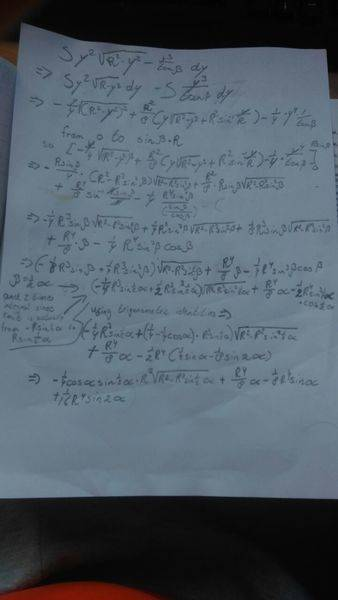So how to simplify this further to r^4/8(a-sin(a))

No one :(?